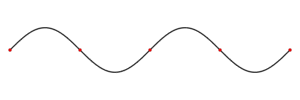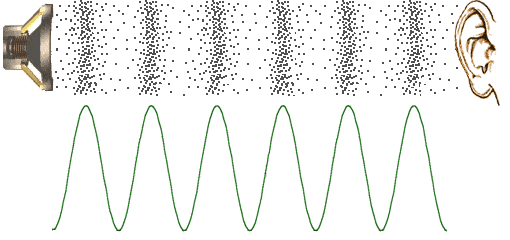# What exactly are waves and how do they look?

• B
Now, whenever I asked this question, my teachers either responded with a diagram of a wave like this (psst! it doesn't move in real life, I didn't go to Hogwarts.):or often referred to the analogy of water waves (when you drop something in water, the wave goes outward).

But when we talk about a particle, like an electron, being a wave, or sound waves, what exactly does that mean? Sound waves, as far as know, compress and contract air particles and we represent that contraction and compression via waves-- wherever compression is high, we have the wave more in the negative axis (or any other convention that we might choose). Is this correct?

What about a particle being a wave? Neither is there a sea of particles which bounce in their respective places which gives us the graph of a wave, nor are electrons emitted out of their orbits via which, we'd measure the frequency of their emission and represent it in a graph. So, what exactly does it mean for a particle being a wave, or for that matter, sound?

hilbert2
Gold Member
A wave is a disturbance in some field, and a field can be any function of position and time coordinates. Sound waves are a disturbance in the air pressure field, and waves on water are disturbances of the surface topography of the water (the function which gives the deviation of the local surface level from the level of still water).

All fields have some kind of an equation of motion. Those equations are called field equations. Often these equations are linear partial differential equations, and have solutions that have the form of sine and cosine functions, like the animated image in your post.

The wave in quantum mechanics represents the probability amplitude for finding the particle at that position.

The wave in quantum mechanics represents the probability amplitude for finding the particle at that position.So, like sound waves that are given above, I should think of a similar picture for a particle; but instead of actual air particles like in the following image, I should imagine the denser regions where there's a higher probability of that particle being present and the rarer regions where there's a lower probability, correct?

•edguy99
jtbell
Mentor
"Probability amplitude" is not the same thing as "probability [distribution]". The wave of probability amplitude for an idealized particle with a fixed well-defined momentum is a complex wave: ##\Psi(x,t) = Ae^{i(kx - \omega t)}##. The corresponding probability distribution is ##\Psi^*\Psi = A^*A## which is a constant with no "peaks" or "troughs". The particle is equally likely to be found at any position x.

"Probability amplitude" is not the same thing as "probability [distribution]". The wave of probability amplitude for an idealized particle with a fixed well-defined momentum is a complex wave: ##\Psi(x,t) = Ae^{i(kx - \omega t)}##. The corresponding probability distribution is ##\Psi^*\Psi = A^*A## which is a constant with no "peaks" or "troughs". The particle is equally likely to be found at any position x.
I'm extremely sorry, but I did not understand any of that, I've graduated only high school. Is there a simpler language?

•ProfuselyQuarky
Phys12, the nodes would correspond to the areas of low distribution probability so your green line needs fewer peaks and troughs. The amplitude (whether negative or positive) would correspond to the probability amplititude. Square the amplitude to get the probability.

bhobba
Mentor
But when we talk about a particle, like an electron, being a wave, or sound waves, what exactly does that mean?

It means its wrong.

Its a half truth we tell beginning students because they need some place to start.

The best that can be said is in some circumstances we have solutions that are mathematically like waves.

Don't worry about it for now. As you progress in QM the issue will be rectified.

Thanks
Bill

edguy99
Gold Member
To get started, one way to visualize a wave is a flying harmonic oscillator. A simple harmonic oscillator is anything with a linear restoring potential. The simplest examples are things like a spring, or a string with tension, an expanding and contracting bubble, or a water wave. In 1926, Erwin Schrödinger, described mathematically how a harmonic oscillator stores energy and how to calculate how much energy it stores. These same calculations (or extensions of the same idea) are used to find all sorts of things from the energy of a photon (depends on its wavelength) to how an electron will interact with a grid of nickel atoms (it flies off in slightly different direction in a way the depends on its wavelength).

A visual example of this idea is here: http://www.animatedphysics.com/games/photon_cavity.htm